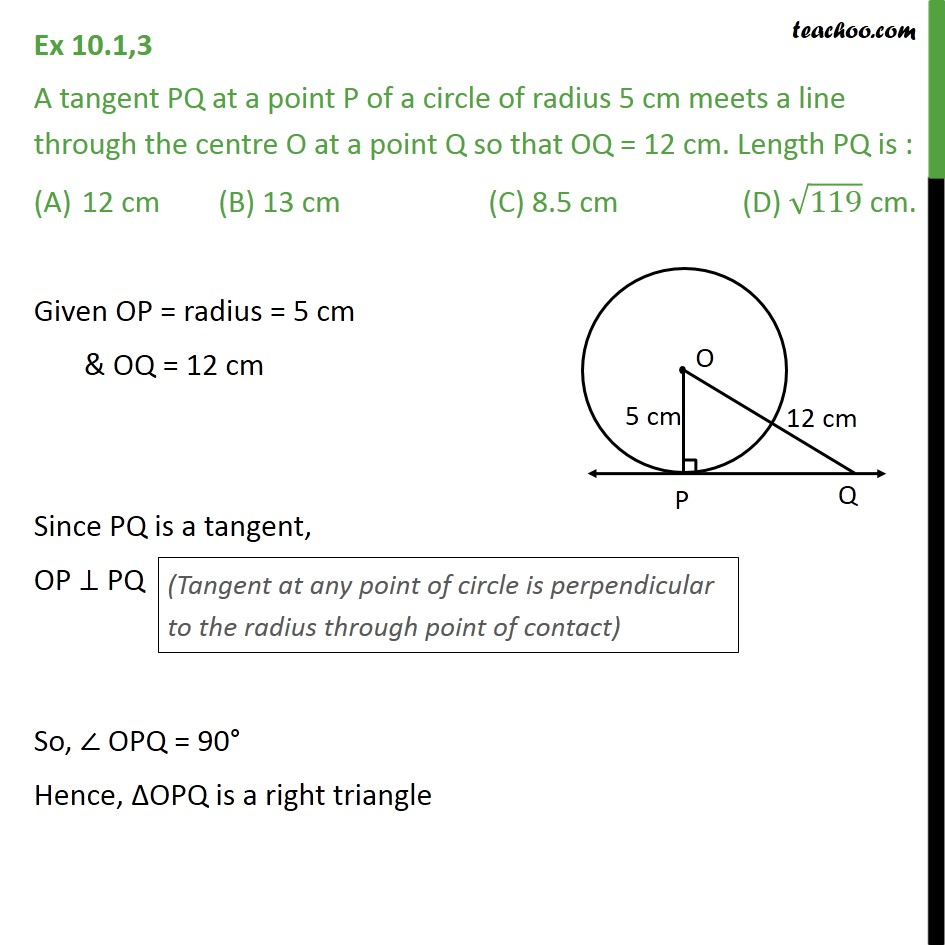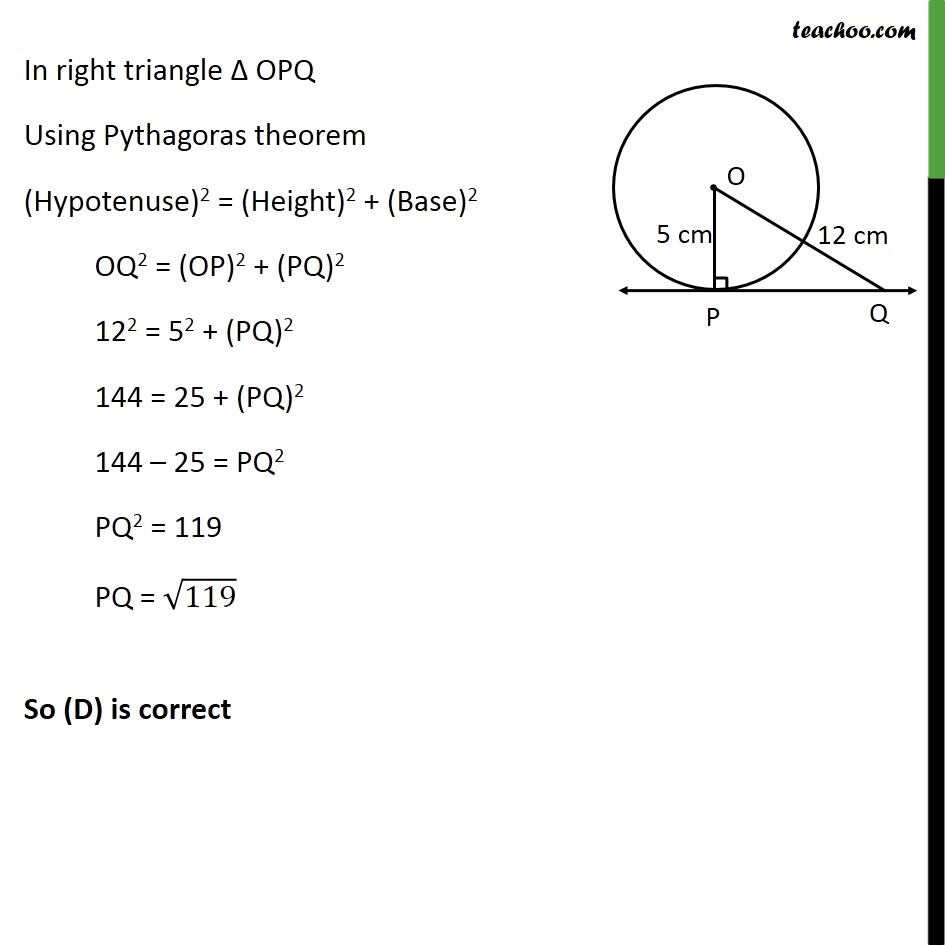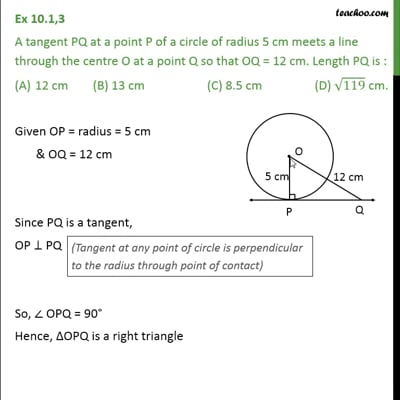Theorem 10.1: Tangent perpendicular to radius (numerical type)This video is only available for Teachoo black users

Introducing your new favourite teacher - Teachoo Black, at only ₹83 per month

### Transcript

Ex 10.1,3 A tangent PQ at a point P of a circle of radius 5 cm meets a line through the centre O at a point Q so that OQ = 12 cm. Length PQ is : 12 cm (B) 13 cm (C) 8.5 cm (D) √119 cm. Given OP = radius = 5 cm & OQ = 12 cm Since PQ is a tangent, OP ⊥ PQ So, ∠ OPQ = 90° Hence, ΔOPQ is a right triangle In right triangle Δ OPQ Using Pythagoras theorem (Hypotenuse)2 = (Height)2 + (Base)2 OQ2 = (OP)2 + (PQ)2 122 = 52 + (PQ)2 144 = 25 + (PQ)2 144 – 25 = PQ2 PQ2 = 119 PQ = √119 So (D) is correct